Name:    Quiz 7.1-7.4

Multiple Choice
Identify the choice that best completes the statement or answers the question.

Write the word sentence as an equation.

1.

The sum of a number c and 5 is equal to 10.
 a. c + 5 = 10 + 5 c. c + 10 = 5 b. c + 5 = 10 d. 5 + 10 = c

2.

The quotient of a number c and 10 equals 5.
 a. 10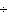c = 5 c. c10 = 5 b. c10 = 5c d. c5 = 10

Write an equation that can be used to find the value of x.

3.

Area of triangle: 36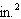a.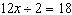c.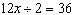b.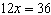d.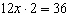Name the inverse operation you can use to solve the equation.

4.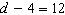a. subtraction c. division b. addition d. multiplication

Solve the equation.  Check your solution.

5.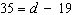a. 16 c. 54 b. 51 d. 57

6.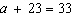a. 11 c. 10 b. 56 d. 43

7.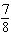=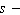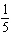a.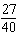c.b.d.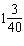Solve the equation. Check your solution.

8.

15.8 =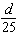a. 395 c. 9.2 b. 40.8 d.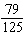9.

Parallelogram
Area = 238 square units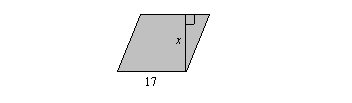a. 15 units c. 14 units b. 102 units d. 17 units

Solve the equation. Check your solution.

10.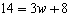a. 2 c. 18 b.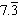d. 3

11.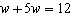a. 6 c. 2 b. 3 d. 72

12.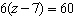a. 612 c. 17 b. 10 d. 102

Numeric Response

1.

What number divided by 16 equals 1?

Solve the equation. Check your solution.

2.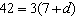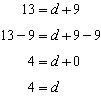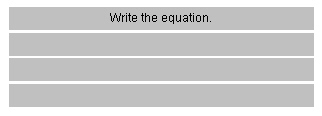What property of equality would you use to solve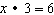?